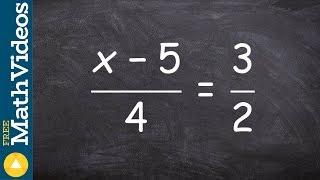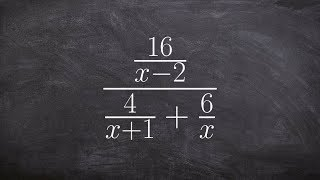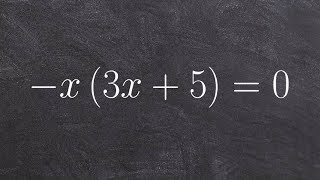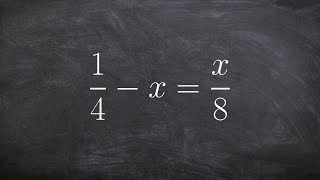Try NerdPal! Our new app on iOS and Android

# Solve the product $\left(x+1\right)\left(x-4\right)$

## Related Videos

Go!
Go!
1
2
3
4
5
6
7
8
9
0
a
b
c
d
f
g
m
n
u
v
w
x
y
z
.
(◻)
+
-
×
◻/◻
/
÷
2

e
π
ln
log
log
lim
d/dx
Dx
|◻|
θ
=
>
<
>=
<=
sin
cos
tan
cot
sec
csc

asin
acos
atan
acot
asec
acsc

sinh
cosh
tanh
coth
sech
csch

asinh
acosh
atanh
acoth
asech
acsch

### Videos### Learn how to apply the cross product to solve the proportion ex 10, (x - 5)/4 = 3/2### Polynomial special products: difference of squares | Algebra 2 | Khan Academy### Tutorial - Simplifying complex fractions ex 37, (16/(x-2) / (4/x+1)+6/x)### Algebra 2 - Solving a factored quadratic equation using the zero product property, -x(3x + 5) = 0### Equations with rational expressions | Mathematics III | High School Math | Khan AcademySnapXam A2

### beta Got another answer? Verify it!

Go!
1
2
3
4
5
6
7
8
9
0
a
b
c
d
f
g
m
n
u
v
w
x
y
z
.
(◻)
+
-
×
◻/◻
/
÷
2

e
π
ln
log
log
lim
d/dx
Dx
|◻|
θ
=
>
<
>=
<=
sin
cos
tan
cot
sec
csc

asin
acos
atan
acot
asec
acsc

sinh
cosh
tanh
coth
sech
csch

asinh
acosh
atanh
acoth
asech
acsch

$\left(x+1\right)\left(x-4\right)$